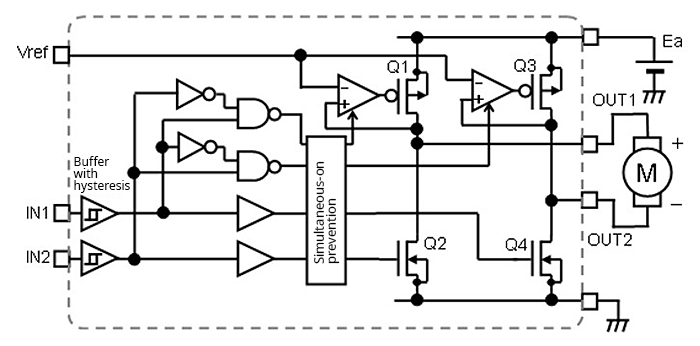# Driving Brushed DC Motors with an H-Bridge:High-Side Voltage Linear Control

2022.07.06

・A circuit is configured to enable linear control of the H-bridge circuit high-side voltage.

・By controlling the H voltages of OUT pins, linear control of the rotation rate/torque of the motor is possible.

Starting with this article, a number of methods for driving brushed DC motors using an H-bridge circuit are explained.

## Driving Brushed DC Motors with an H-Bridge:High-Side Voltage Linear Control

In this driver circuit example, the voltage applied to a motor is controlled with the H voltage of the OUT terminals made variable, by linear control of high-side P-channel MOSFETs. The H voltage of the OUT terminals is controlled by the DC voltage applied to the Vref terminal; theoretically the voltage is the same as the voltage applied to the Vref terminal. As a result, the motor rotation rate/torque can be controlled.In this example, when the H-bridge switching control logic turns on the high-side MOSFETs (Q1, Q3), op-amps apply a bias to the high-side MOSFET gates to turn on the MOSFETs, and the MOSFET drain voltages are fed back to the non-inverting inputs of the op-amps. In accordance with the principles of op-amp feedback circuits, in this circuit, feedback control is performed such that the voltages at the inverting input and the non-inverting input are equal. In this example, the voltage applied to the Vref pin, which is the voltage at the op-amp’s inverting input terminal, is equal to the H voltage of the OUT terminal, which is the MOSFET drain voltage and the voltage at the op-amp’s non-inverting input terminal. The gain of this feedback circuit is +1, and therefore the voltage ratio of Vref to OUT is 1:1.

The reader may find it strange that feedback is applied to the non-inverting input terminal; but because a P-channel MOSFET is active-low, the inverted voltage of the op-amp output is fed back. The general idea is the same as for a feedback circuit that uses PNP transistors as boosters.

The operation of the H-bridge control circuit is the same as that explained in “Switching Output States”.